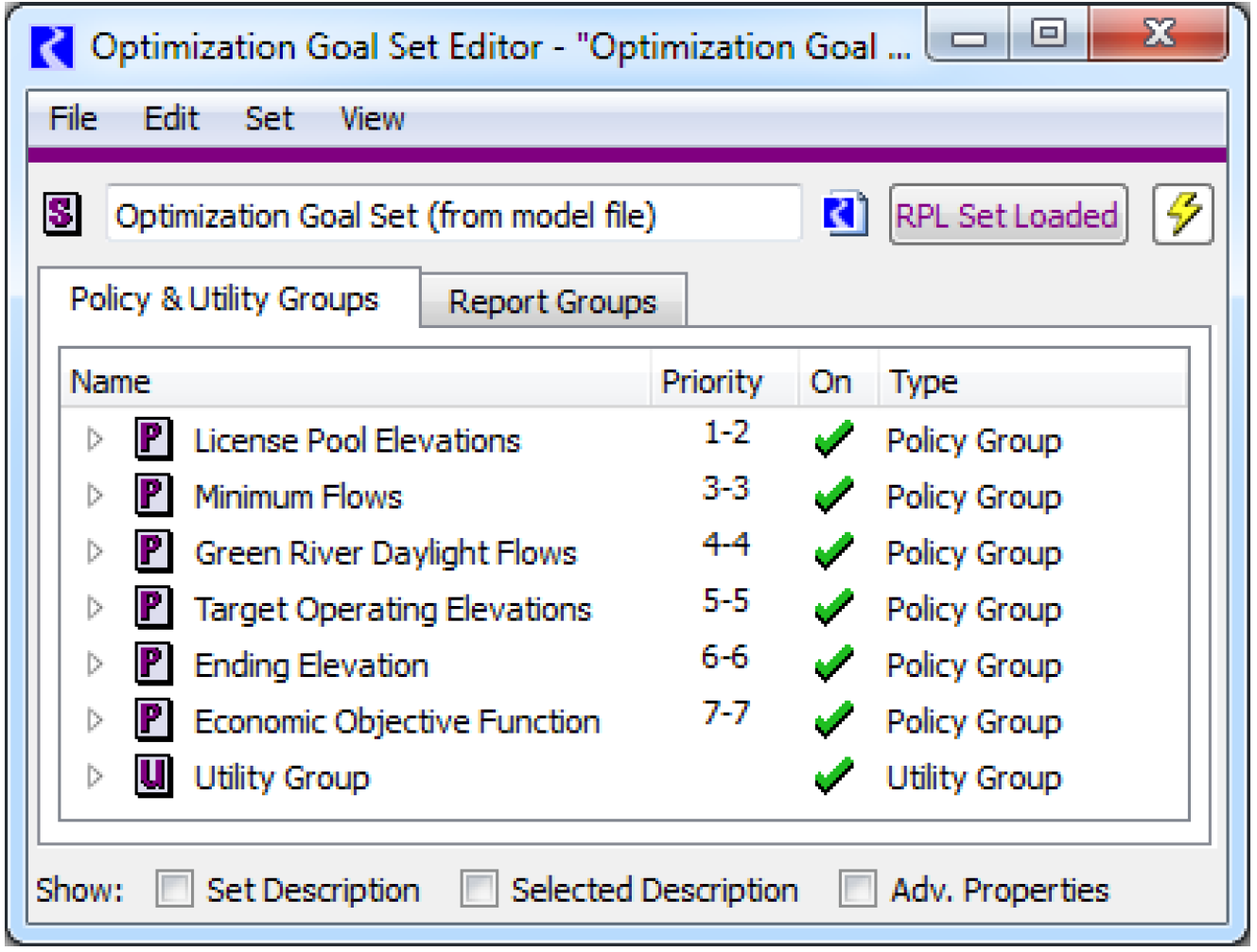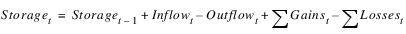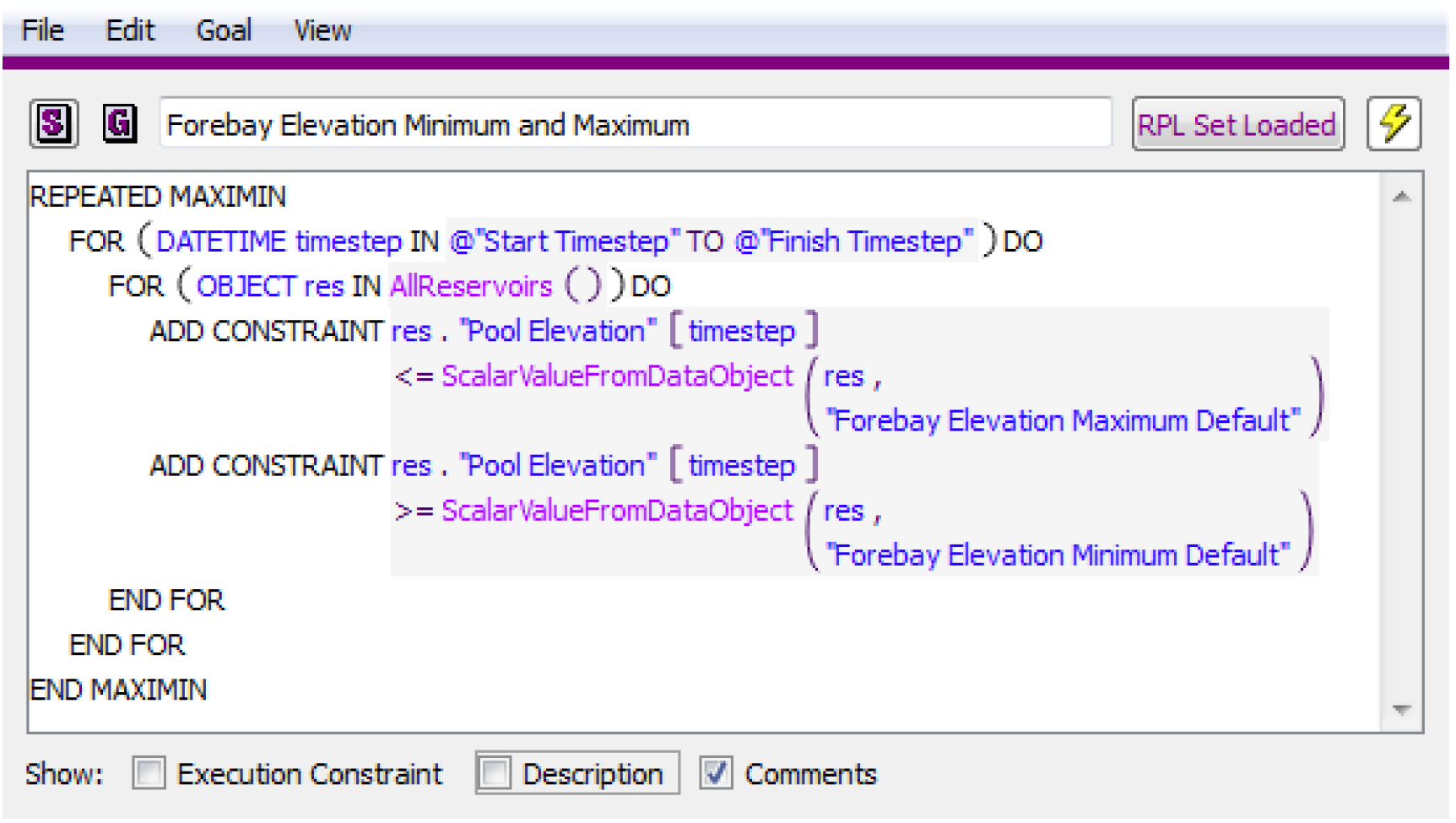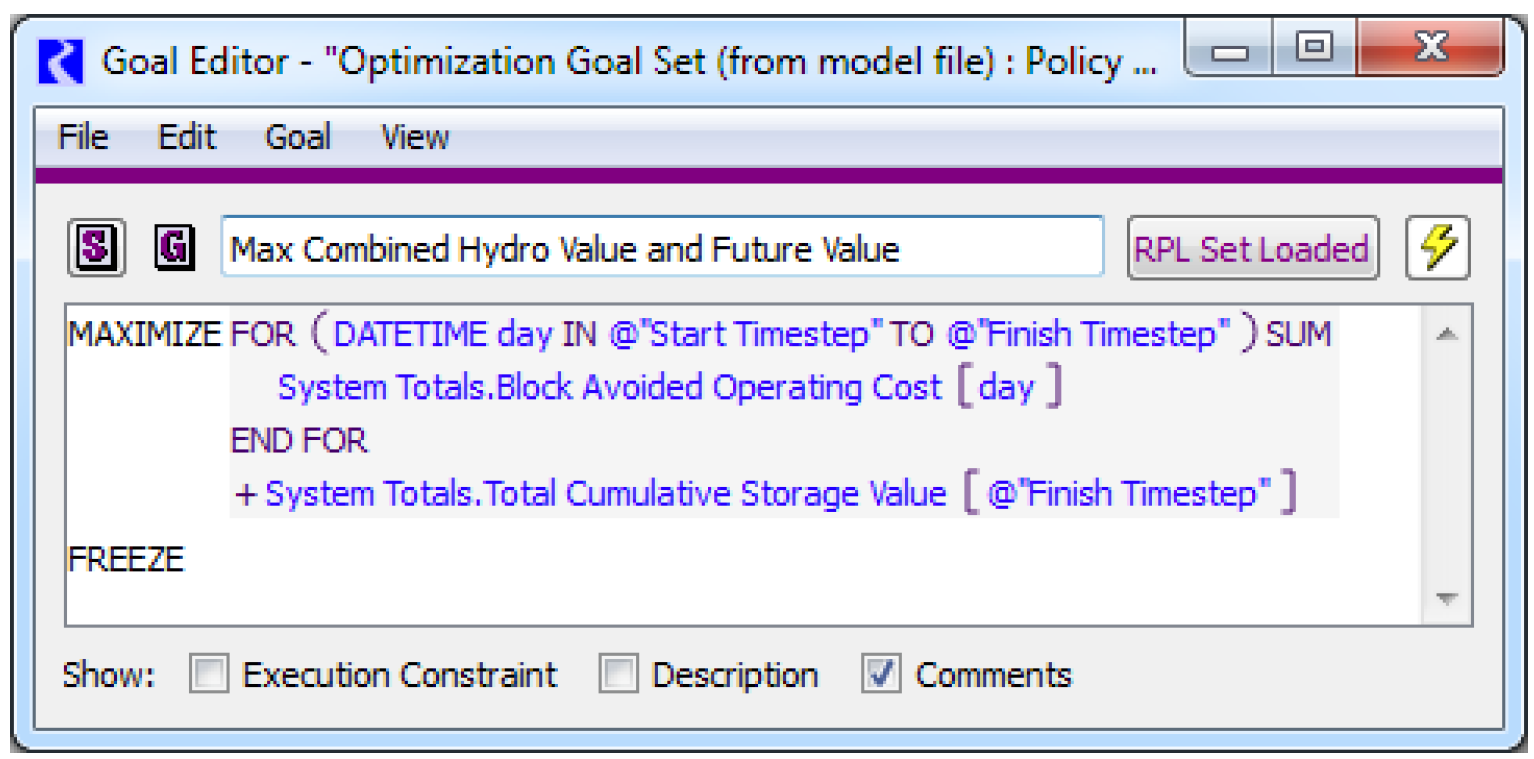Optimization : Optimization Overview : About Optimization
The RiverWare Optimization solver provides a different solution approach. Instead of solving a model on a timestep-by-timestep basis, Optimization provides a single global solution over all objects and all timesteps. Typically, Optimization is used to determine the best solution in terms of a stated objective, such as maximizing the value of hydropower over the run period, while also satisfying higher-priority policy constraints.
This document provides the following information about the RiverWare Optimization solver.
• Overview of the Optimization solver
• Details about the preemptive linear goal programming approach used by RiverWare
• How Optimization interacts with Simulation and Rulebased Simulation
• How variables and constraints (policy) are formulated for RiverWare Optimization
• Methods and slots on each object that apply for Optimization
• Creating and analyzing an Optimization model
• Debugging an Optimization model
• Technical information about units, scale, and mixed-integer programming
Following are key characteristics of Optimization:
System-wide Approach
Simulation and Rulebased Simulation solve each object individually, one timestep at a time. Optimization, on the other hand, provides a global solution across all objects and across all timesteps. This allows the solution to trade off objectives both spatially and over time.
For example, using Optimization, the model can look ahead to a peak price period and send water from upstream reservoirs to a downstream reservoir with high generating capacity early in the run, so that it can generate at maximum capacity during peak prices. At the same time, it ensures that the upstream reservoirs do not release so much water that they will violate their elevation targets by the end of the run based on inflow forecasts.
Hydropower objectives are a common focus of Optimization models. RiverWare includes some built-in functionality that assists in formulating common hydropower objectives; however, there is nothing that limits RiverWare Optimization objectives to be related to hydropower, nor is hydropower required to be included in an Optimization model. Optimization models can just as easily optimize a water management objective unrelated to hydropower.
Preemptive Linear Goal Programming
The Optimization solution in RiverWare is a preemptive linear goal program. The user expresses the policy of the system in a set of prioritized goals. These goals contain either constraints or objectives. A linear program (LP) is solved at each priority level, beginning at the highest priority. If the goal contains a set of soft constraints, the objective function for the LP is to maximize the satisfaction of the constraints. The objective value (level of satisfaction) will not be reduced when the LPs are solved for lower priority goals. In other words, once a constraint has been satisfied at a high priority, it will not be violated to meet a lower priority constraint or objective. In this way, as the solution progresses through the priorities, it shrinks the solution space or removes degrees of freedom from the solution. Typically the final priority is a maximize or minimize objective, such as maximizing the total value of hydropower over the run period. The objective will be maximized/minimized within the reduced solution space after maximizing the satisfaction of all higher priority constraints.
Figure 1.1RiverWare provides three solution approaches in the case that not all soft constraints within a goal cannot be satisfied due to hydrologic conditions and/or higher priority constraints. The first approach, Summation, minimizes the total deviation from the constraint values. The second approach, Maximin, minimizes the single largest deviation (technically it maximizes the lowest satisfaction). The third approach, Repeated Maximin, minimizes the single largest deviation. Then it freezes that value and minimizes the next largest deviation, and so on until it has either minimized all deviations or satisfied all remaining constraints. In practice, the Repeated Minimax approach is most-often used.
Optimization Variables
An optimization problem consists of a set of equations that include linear combinations of optimization variables. In RiverWare, some Optimization variables are directly related to slots. For example, Outflow and Storage on a reservoir object are common variables. Each of these slots at each timestep corresponds directly to an individual variable in the Optimization problem. In some cases slots correspond to a linear combination of variables. For example, Power is typically defined by a piecewise linear function of Turbine Release. Each individual variable, or piece, is multiplied by the slope of that segment of the power curve. The sum of the pieces is equal to the total Turbine Release. Some variables have no relation to a slot in RiverWare. For example, the satisfaction level of each Repeated Maximin iteration is added to the Optimization problem as a variable. RiverWare decides automatically which variables to add to the Optimization problem based on what is referenced in the Optimization Goal Set.
Physical Constraints
RiverWare automatically adds all relevant physical constraints to the Optimization problem. For example, the mass balance constraint for a reservoir isThis is the defining constraint for the Storage, Inflow and Outflow variables at time t. RiverWare determines which individual variables to include in Gains and Losses depending on the method selection on the reservoir. For example, Evaporation could be an additional loss variable in the mass balance constraint. For a reach, the defining physical constraints are determined by the selected routing method.
RiverWare only adds physical constraints as necessary based on the policy in the Optimization Goal Set. In this way it minimizes the size of the Optimization problem in order to achieve maximum computational efficiency. Physical constraints are always treated as hard constraints.
Prioritized Policy
The policy of a river basin is expressed by the user in an Optimization Goal Set. Like rulesets in Rulebased Simulation, the Goal Set is formulated in RiverWare Policy Language (RPL). Also similarly to a ruleset, the goals in a Goal Set are expressed in priority order. For example, license minimum and maximum pool elevation constraints for reservoirs might be the highest priority goal. These are goals that would essentially never be violated. Lower priority goals might include target operating elevation range constraints. These would represent elevations that should be maintained under normal operating conditions but might be exceeded in extreme scenarios such as flood control operations. Goals with a Minimize or Maximize objective tend to be at the lowest priority, after all policy constraints have been satisfied to the greatest extent possible.
A user has the flexibility to formulate any type of constraint or objective they desire as long as the expressions are linear combinations of variables. Constraints can include average values over multiple timesteps or summations over multiple slots on multiple objects. Similar constraints for multiple objects can be grouped into a single constraint statement. For example, a single maximum elevation constraint can be added for all reservoirs in a model by creating a loop over a list of reservoirs and referencing the maximum elevations from a data object slot corresponding to each reservoir.
Figure 1.2Conditional statements can be added for the inclusion of constraints in the form of If-Then statements. For example, seasonal constraints might apply only if the run period is within specified dates, or Special Operations constraints might only apply if data are present in a certain slot.
Objectives allow for a trade-off between present value and future value. An objective might include the combined value of the hydropower generated during the run and the value of energy in storage (future potential value) at the end of the run.
Figure 1.3Revised: 08/02/2021